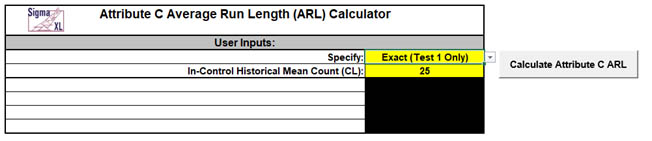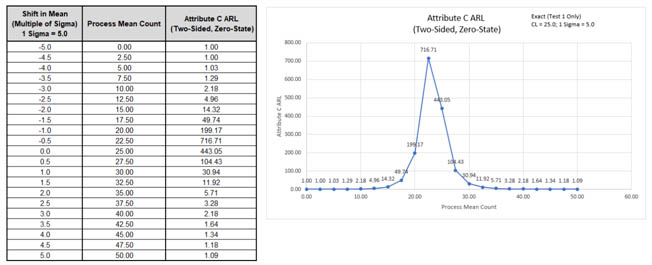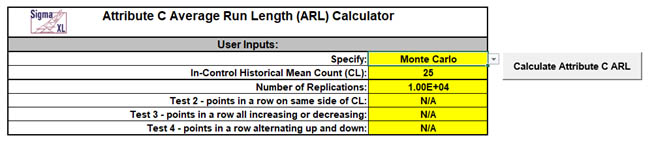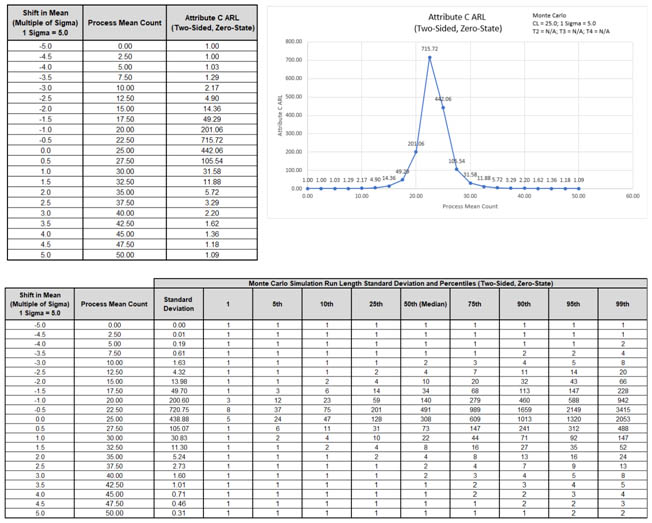Include Top

# Attribute C ARL

1. Click SigmaXL > Templates & Calculators > Control Chart Templates > Average Run Length (ARL) Calculators >Attribute C ARL. This template is also located at SigmaXL > Control Charts > Control Chart Templates> Average Run Length (ARL) Calculators >Attribute C ARL.

2. The default template settings are Specify = Exact (Test 1 Only), In-Control Historical Mean Count (CL) = 25.Notes: Specify Exact (Test 1 Only) or Monte Carlo using the drop-down list. Parameters to be specified will be shown in yellow highlight, otherwise they are hidden. Exact uses the Poisson cumulative distribution function. Monte Carlo simulation uses Poisson random data with specified proportion and allows you to assess the ARL performance of all 4 Tests for Special Causes. Test 1 - 1 point more than 3 standard deviations from the center line (CL) is always applied. Monte Carlo simulation also produces the table of Run Length Standard Deviation and Percentiles (scroll right to view).

3. Click the Calculate Attribute C ARL button to reproduce the ARL table and chart.The ARL0 (in-control ARL with 0 shift in mean) for the Attribute C chart is 443.05. The ARL1 for a small positive 1 sigma shift in mean count is 30.94, so is slow to detect. On the other hand, a large 3 sigma shift in mean count has an ARL = 2.18, so is detected rapidly. Small negative shifts of one-half or one sigma cannot be detected.

Note: The Process Mean Count values are displayed on the ARL chart, but the shift in mean count as a multiple of sigma is also included in the table.

4. Now we will use Monte Carlo simulation to obtain approximate Run Length standard deviation and percentiles for the Attribute C chart. Select Specify = Monte Carlo. Enter In-Control Historical Mean Count (CL) = 25, Number of Replications = 1e4, Test 2 = N/A, Test 3 = N/A, Test 4 = N/A.5. Click the Calculate Attribute C ARL button to produce the Monte Carlo approximate ARL table, ARL chart and Run Length Standard Deviation and Percentiles table (scroll right to view). Monte Carlo simulation with 10,000 (1e4) replications will take about a minute to run.The additional run length statistics show the large variation of run length values. The MRL0 = 308 (in-control median run length with 0 sigma shift in process mean count).

Note: The results will vary slightly since this is Monte Carlo simulation

6. Now we will use Monte Carlo simulation to assess the Attribute C chart with all 4 Tests for Special Causes applied. Enter Specify = Monte Carlo, In-Control Historical Mean Count (CL) = 25. Number of Replications = 1e4, Test 2 = 9, Test 3 = 6, and Test 4 = 14.Note: These are the test settings used as defaults in SigmaXL > Control Charts > ‘Tests for Special Causes’ Defaults. Test 1 is always applied.

7. Click the Calculate Attribute C ARL button to produce the Monte Carlo approximate ARL table, ARL chart and Run Length Standard Deviation and Percentiles table.ARL0 with all 4 tests for special causes is approx. 151.4. This is a poor performance with a 2.9 x increase (443.1/151.4) in false alarms compared to Exact Test 1 only. MRL0 is approx. 106. On the other hand, ARL1 for a small 1 sigma shift in proportion is approx. 15.1, so is much faster to detect than the Exact Test 1 only ARL1 of 30.94. It is now possible to detect a small negative one sigma shift in mean count.

# Template Notes:

1. Specify Exact (Test 1 Only) or Monte Carlo using the drop-down list. Parameters to be specified will be shown in yellow highlight, otherwise they are hidden.
2. Exact uses the Poisson cumulative distribution function. Monte Carlo simulation uses Poisson random data with specified proportion and allows you to assess the ARL performance of all 4 Tests for Special Causes. It also produces the table of Run Length Standard Deviation and Percentiles (scroll right to view).
3. Test 1 - 1 point more than 3 standard deviations from the center line (CL) is always applied.
4. Enter the Subgroup Size.
5. Enter the In-Control Historical Mean Count (CL).
6. If applicable, enter Number of Replications. 1000 (1e3) replications will be fast, approx. 10 seconds, but will have an ARL0 error approx. = +/- 10%; 10,000 (1e4) replications will take about a minute, with an ARL0 error = +/- 3.2%; 100,000 (1e5) replications will take about ten minutes, with an ARL0 error = +/- 1%.
7. If applicable, select values for Tests 2 to 4 using the drop-down list. "N/A" indicates that the test is not applied. Tests 2 and 3 provide options that match those provided in SigmaXL's 'Tests for Special Causes' Defaults dialog.
8. Click the Calculate Attribute C ARL button to produce the ARL table and chart. If Monte Carlo was selected, the table of Run Length Standard Deviation and Percentiles will also be produced.
9. The Attribute C ARL is for a two-sided chart with zero-state, i.e., the shift is assumed to occur at the start. The proportion is also assumed to be known. This will not likely be the case in use, but is still useful for determining parameter settings and comparison of ARL across chart types.
10. The ARL Chart is similar to an Operating Characteristic (OC) Curve, except that the Y axis is ARL rather than Beta probability. Note that the C chart is ARL biased for small mean counts (<40), with=with maximum=maximum ARL=ARL occurring=occurring below=below the=the CL.=CL.
11. Due to the complexity of calculations, SigmaXL must be loaded and appear on the menu in order for this template to function. Do not add or delete rows or columns in this template.

# REFERENCES:

1. Montgomery, D.C. (2013), Introduction to Statistical Quality Control, Seventh Ed., Wiley.

# Web Demos

Our CTO and Co-Founder, John Noguera, regularly hosts free Web Demos featuring SigmaXL and DiscoverSim